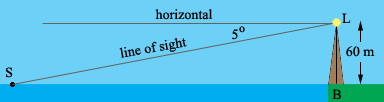SEARCH HOMEMath Central Quandaries & QueriesQuestion from Chelsey, a student: I have a question in regards to how do I know when to use tangent or cosine when determining angles. The question is: Looking north from the observation deck of a lighthouse 60 m above the sea, a lighthouse keeper sees two ships. The angles of depression to the ships is 5 degrees and 10 degrees. How far apart are the ships? I don't understand which one to use when solving the equation.Hi Chelsey,

I would always draw a diagram.S is a ship, L is the top of the lighthouse and B is its base. The angle of depression, that is the angle between the line of sight and horizontal is 5o. Since the surface of the water is parallel to the line labeled horizontal the measure of the angle LSB is 5o also. Thus you have a right triangle LSB, you know the measure of the angle LSB, the length of the side opposite angle LSB (60 m) and you want to know the length of the side SB which is adjacent to the angle LSB. The trig function that relates the angle, the opposite side and the adjacent side is the tangent. Thus it's the tangent function you want for this problem.

I hope this helps,
PennyMath Central is supported by the University of Regina and The Pacific Institute for the Mathematical Sciences.